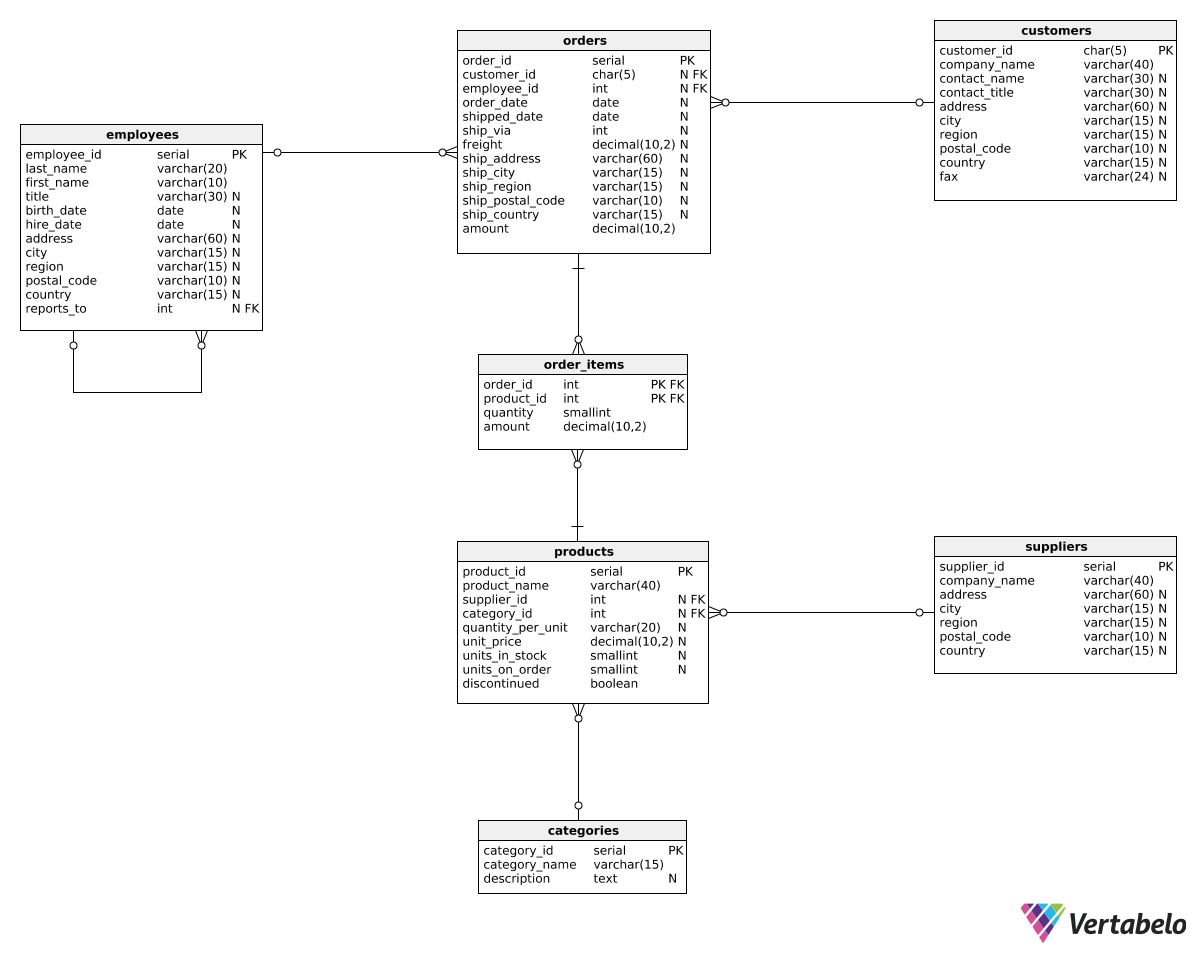Deals Of The Week - hours only!Up to 80% off on all courses and bundles.-Close
Introduction
Total revenue for multiple periods
Calculating deltas
Creating "revenue in quarters" reports
Summary

## Instruction

Good job! Now that we know how to calculate the revenue value for the previous period, we can easily calculate the revenue change (delta) between two periods. Take a look:

SELECT
EXTRACT(year FROM order_date) AS revenue_year,
SUM(amount) AS total_revenue,
SUM(amount) - LAG(SUM(amount), 1) OVER (ORDER BY EXTRACT(year FROM order_date)) AS delta
FROM orders
GROUP BY EXTRACT(year FROM order_date)
ORDER BY EXTRACT(year FROM order_date);


Result:

revenue_year total_revenue delta
2016 208083.98 null
2017 617085.21 409001.23
2018 440623.90 -176461.31

In the delta column, we simply subtracted the revenue generated in the previous year from the revenue generated in the current year. Once again, we used the LAG() function pattern from the previous exercise.

## Exercise

Show the total revenue for each quarter in each year alongside the delta (revenue change) as compared to the previous quarter. Show the following columns: revenue_year, revenue_quarter, total_revenue, and delta.

In the first row, leave the delta value as NULL. Order the rows by year and quarter.

### Stuck? Here's a hint!

Modify the query from the explanation. Use EXTRACT(year FROM order_date) and EXTRACT(quarter FROM order_date) in the SELECT, OVER(), and GROUP BY clauses.